# Markov Decision Process (MDP)

## 1 Markov Decision Processes简介

Markov decision processes (MDPs) provide a mathematical framework for modeling decision making in situations where outcomes are partly random and partly under the control of a decision maker.

### 1.1 一个例子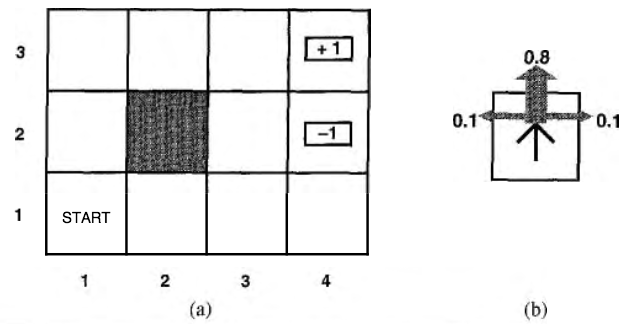Figure 1: (a) 一个简单的 $$4 \times 3$$ 环境，(b) 这个环境的转移模型示意图

1、初始状态： $$s_0$$
2、转移模型： $$T(s, a, s')$$
3、回报函数： $$R(s)$$

2 (a)是对于非终止状态中 $$R(s) = -0.04$$ 的随机环境的一个最优策略。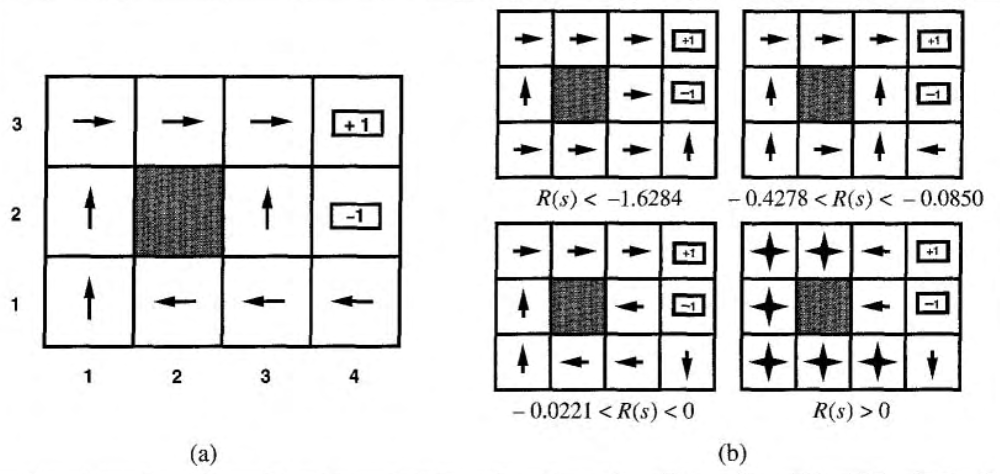Figure 2: (a) 是对于非终止状态中 $$R(s) = -0.04$$ 的随机环境的一个最优策略，(b) 对于不同的 $$R(s)$$ 对应的最优策略。

2 (a)是对于图 1 环境的一个最优策略。注意，因为多走一步的代价与偶然结束在状态 $$(4, 2)$$ 中的惩罚相比是相当小的，所以状态 $$(3,1)$$ 的最优策略是谨慎的：策略建议绕一个远道，而不走可能进入状态 $$(4, 2)$$ 的近道，即当处于 $$(3,1)$$ 时我们往左走，而不往上走。当非终止状态 $$R(s) < -1.6284$$ 时，智能体觉得“生活太痛苦”，于是直奔最近的出口，即使出口的价值是 $$-1$$ ；当非终止状态 $$-0.4278 < R(s) < -0.0850$$ 时，智能体觉得“生活很不愉快”，选择通往 $$+1$$ 状态的最近路径，并愿意冒偶然落进 $$-1$$ 状态的风险，如当处于 $$(3,1)$$ 时智能体往上走，而不往左走；当非终止状态 $$-0.0221 < R(s) < 0$$ 时，智能体觉得“生活只是有些沉闷”，最优策略选择根本不冒险。最后，如果非终止状态 $$R(s) > 0$$ ，则智能体觉得“生活令人愉快”，智能体会躲避所有的出口。

### 1.2 “累加回报”和“折扣回报”

$V([s_0, s_1, s_2, \cdots]) = R(s_0) + R(s_1) + R(s_2) + \cdots$

$V([s_0, s_1, s_2, \cdots]) = R(s_0) + \gamma R(s_1) + \gamma^2 R(s_2) + \cdots$

$E[R(s_0) + \gamma R(s_1) + \gamma^2 R(s_2) + \cdots]$

\begin{aligned} \pi^* & = \operatorname{arg} \underset{\pi}{\max} E[R(s_0) + \gamma R(s_1) + \gamma^2 R(s_2) + \cdots \mid \pi, s_0 = s] \\ & = \operatorname{arg} \underset{\pi}{\max} E \left[\sum_{t=0}^{\infty} \gamma^t R(s_t) \mid \pi, s_0=s \right] \\ \end{aligned}

### 1.3 用MDP对“给西瓜浇水问题”进行建模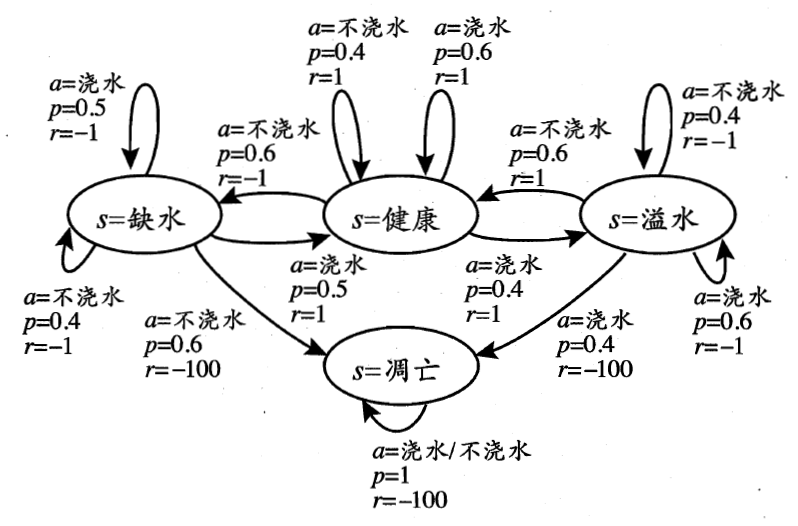Figure 3: “给西瓜浇水问题”的马尔可夫决策过程

3 给出了一个简单的例子：给西瓜浇水的马尔可夫决策过程。该任务中只有四个状态（健康、缺水、溢水、凋亡）和两个动作（浇水、不浇水），在每一个步转移后，若状态是保持瓜苗健康则获得奖赏 $$1$$ ，瓜苗缺水或溢水奖赏为 $$-1$$ ，这时通过浇水或不浇水可以恢复健康状态，当瓜苗凋亡时奖赏是最小值 $$-100$$ ，且无法恢复。图中箭头表示状态转移，箭头旁的 $$a,p,r$$ 分别表示导致状态转移的动作、转移概率以及返回的奖赏。容易看出，最优策略在“健康”状态选择动作“浇水”、在“溢水”状态选择动作“不浇水”、在“缺水”状态选择动作“浇水”、在“凋亡”状态可选择任意动作。

## 2 求解MDP

### 2.1 Value iteration (a.k.a. dynamic programming)

#### 2.1.1 状态的效用值

$V^{\pi}(s) = E[R(s_0) + \gamma R(s_1) + \gamma^2 R(s_2) + \cdots \mid \pi, s_0 = s]$

$V^{\pi^*}(s) = R(s) + \gamma \max_{a \in A}\sum_{s' \in S}p(s' \mid s, a)V^{\pi^*}(s') \tag{Bellman Equation}$

$V^{\pi^*}(s) = \max_{a \in A} \left( R(s, a) + \gamma \sum_{s' \in S}p(s' \mid s, a)V^{\pi^*}(s') \right) \tag{Bellman Equation}$

$\pi^*(s) = \operatorname{arg} \underset{a \in A}{\max} \left( R(s, a) + \gamma \sum_{s' \in S} p(s' \mid s, a) V^{\pi^*}(s') \right)$

#### 2.1.2 求解状态效用值（求解贝尔曼等式）

\begin{align*} V(1,1) = \max \{ & -0.04 + \gamma (0.8 \times V(1,2) + 0.1 \times V(2,1) + 0.1 \times V(1,1)), \tag{UP}\\ & -0.04 + \gamma (0.9 \times V(1,1) + 0.1 \times V(1,2)), \tag{Left}\\ & -0.04 + \gamma (0.9 \times V(1,1) + 0.1 \times V(2,1)), \tag{Down}\\ & -0.04 + \gamma (0.8 \times V(2,1) + 0.1 \times V(1,2) + 0.1 V(1,1)) \} \tag{Right} \end{align*}

$V_{n+1} (s) = \max_{a \in A} \left( R(s, a) + \gamma \sum_{s' \in S} p(s' \mid s, a)V_{n}(s') \right)$

4 是对节 1.1 所示例子进行贝尔曼更新的实例（图片摘自http://mas.cs.umass.edu/classes/cs683/lectures-2010/Lec13_MDP2-F2010-4up.pdf）。Figure 4: 贝尔曼更新的迭代实例（红色数字为迭代次数）

#### 2.1.3 求解最优策略

$\pi^*(s) = \operatorname{arg} \underset{a \in A}{\max} \left( R(s, a) + \gamma \sum_{s' \in S} p(s' \mid s, a) V^{\pi^*}(s') \right)$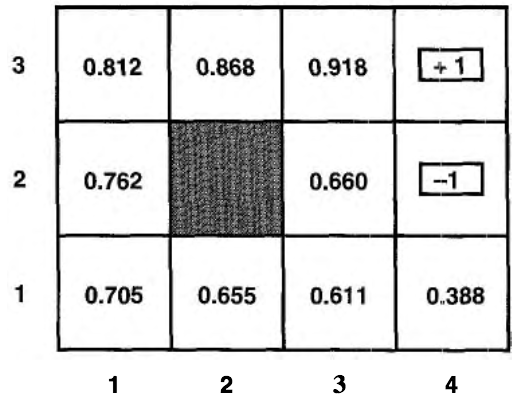Figure 5: 状态效用值

\begin{align*} V(1,1) = \max \{ & -0.04 + \gamma (0.8 \times V(1,2) + 0.1 \times V(2,1) + 0.1 \times V(1,1)), \tag{UP}\\ & -0.04 + \gamma (0.9 \times V(1,1) + 0.1 \times V(1,2)), \tag{Left}\\ & -0.04 + \gamma (0.9 \times V(1,1) + 0.1 \times V(2,1)), \tag{Down}\\ & -0.04 + \gamma (0.8 \times V(2,1) + 0.1 \times V(1,2) + 0.1 V(1,1)) \} \tag{Right} \end{align*}

#### 2.1.4 总结：价值迭代算法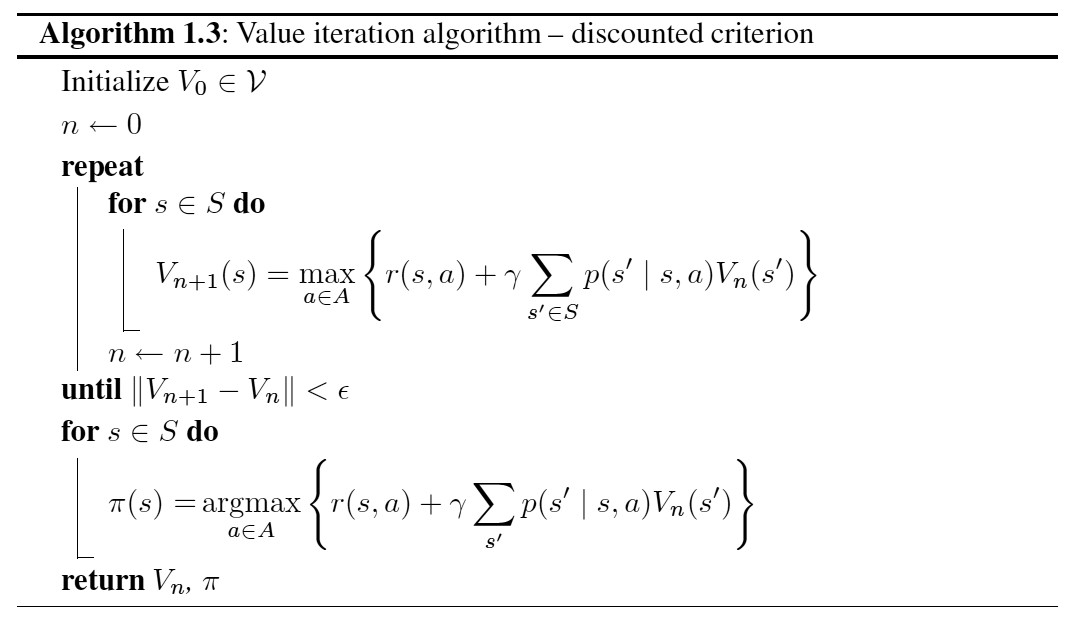Figure 6: 使用“价值迭代”算法求解MDP问题的最优策略

### 2.2 Policy iteration

(1) 策略评价：给定策略 $$\pi_n$$ ，计算在这个策略下每个状态的效用值 $$V_n(s)$$
(2) 策略改进：已知状态效用值，计算新策略 $$\pi_{n+1} = \operatorname{arg} \underset{a \in A}{\max} \left( R(s, a) + \gamma \sum_{s' \in S} p(s' \mid s, a) V_{n}(s') \right)$$

“策略迭代”算法如图 7 所示，这个图中 $$r(s,a)$$ 就是前面介绍的 $$R(s,a)$$ 。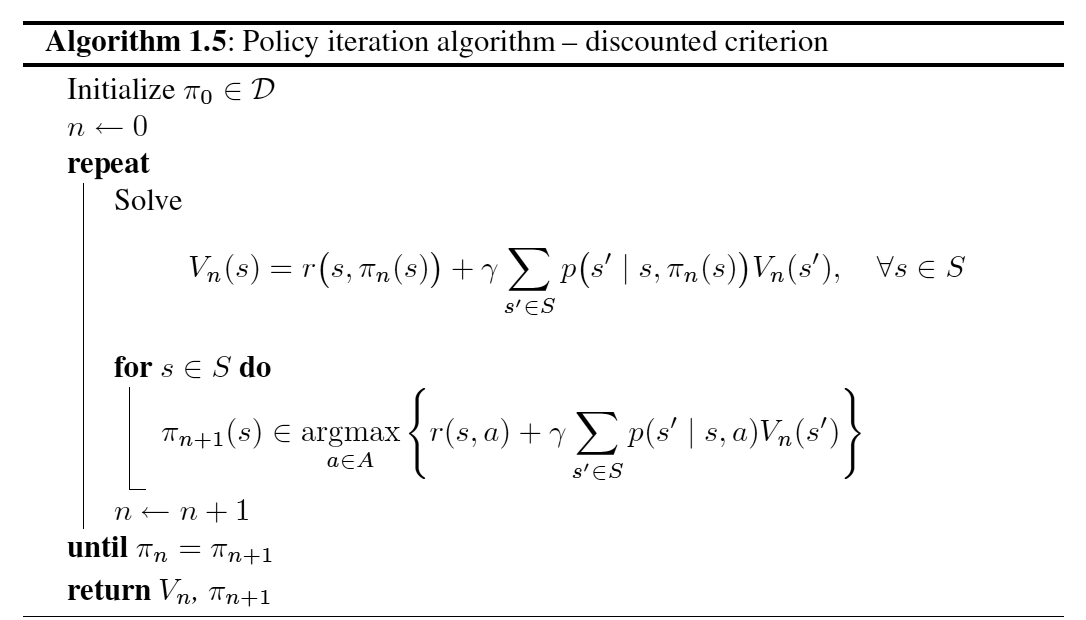Figure 7: 使用“策略迭代”算法求解MDP问题的最优策略

$V_n(s) = R(s, \pi_n(s)) + \gamma \sum_{s' \in S} p(s' \mid s, \pi_n(s)) V_{n}(s')$

\begin{aligned} V_n(1,1) &= 0.8 V_n(1,2) + 0.1 V_n(1,1) + 0.1 V(2,1)\\ V_n(1,2) &= 0.8 V_n(1,3) + 0.2 V_n(1,2) \\ & \cdots \end{aligned}

## 3 参考

《人工智能：一种现代方法》第17章 制定复杂决策
Markov Decision Processes in Artificial Intelligence

Created: <2018-06-02 Sat 00:00>

Last updated: <2018-07-23 Mon 16:31>

Creator: Emacs 25.3.1 (Org mode 9.1.4)